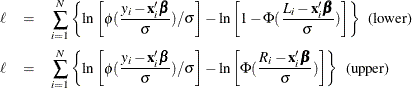The QLIM Procedure

Limited Dependent Variable Models

Subsections:

Censored Regression Models

When the dependent variable is censored, values in a certain range are all transformed to a single value. For example, the standard tobit model can be defined as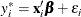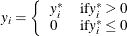where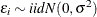. The log-likelihood function of the standard censored regression model is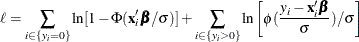where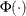is the cumulative density function of the standard normal distribution and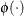is the probability density function of the standard normal distribution.

The tobit model can be generalized to handle observation-by-observation censoring. The censored model on both of the lower and upper limits can be defined as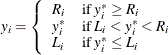The log-likelihood function can be written as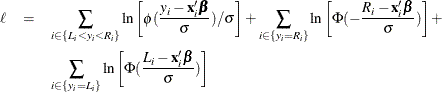Log-likelihood functions of the lower- or upper-limit censored model are easily derived from the two-limit censored model. The log-likelihood function of the lower-limit censored model is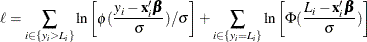The log-likelihood function of the upper-limit censored model is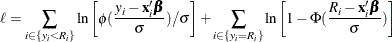Types of Tobit Models

Amemiya (1984) classified Tobit models into five types based on characteristics of the likelihood function. For notational convenience, let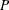denote a distribution or density function,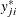is assumed to be normally distributed with mean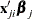and variance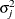.

Type 1 Tobit

The Type 1 Tobit model was already discussed in the preceding section.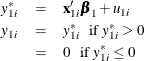The likelihood function is characterized as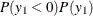.

Type 2 Tobit

The Type 2 Tobit model is defined as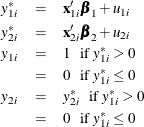where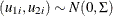. The likelihood function is described as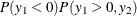.

Type 3 Tobit

The Type 3 Tobit model is different from the Type 2 Tobit in that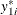of the Type 3 Tobit is observed when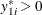.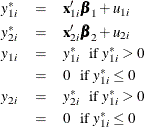where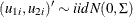.

The likelihood function is characterized as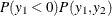.

Type 4 Tobit

The Type 4 Tobit model consists of three equations: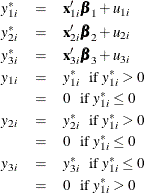where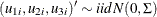. The likelihood function of the Type 4 Tobit model is characterized as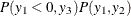.

Type 5 Tobit

The Type 5 Tobit model is defined as follows: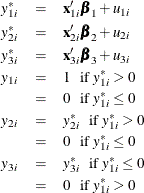where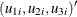are from iid trivariate normal distribution. The likelihood function of the Type 5 Tobit model is characterized as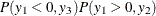.

Code examples for these models can be found in Types of Tobit Models.

Truncated Regression Models

In a truncated model, the observed sample is a subset of the population where the dependent variable falls in a certain range. For example, when neither a dependent variable nor exogenous variables are observed for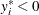, the truncated regression model can be specified.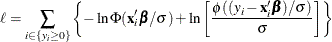Two-limit truncation model is defined as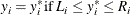The log-likelihood function of the two-limit truncated regression model is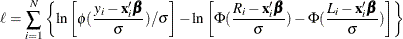The log-likelihood functions of the lower- and upper-limit truncation model are